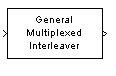# General Multiplexed Interleaver

Permute input symbols using set of shift registers with specified delays

## Library

Convolutional sublibrary of Interleaving

•## Description

The General Multiplexed Interleaver block permutes the symbols in the input signal. Internally, it uses a set of shift registers, each with its own delay value.

This block accepts a scalar or column vector input signal, which can be real or complex. The input and output signals have the same sample time.

The block can accept the data types `int8`, `uint8`, `int16`, `uint16`, `int32`, `uint32`, `boolean`, `single`, `double`, and fixed-point. The output signal has the same data type as the input signal.

## Parameters

Interleaver delay (samples)

A column vector listing the number of symbols that fit into each shift register. The length of this vector is the number of shift registers. (In sample-based mode, it can also be a row vector.)

Initial conditions

The values that fill each shift register at the beginning of the simulation.

If Initial conditions is a scalar, then its value fills all shift registers. If Initial conditions is a column vector, then each entry fills the corresponding shift register. (In sample-based mode, Initial conditions can also be a row vector.) If a given shift register has zero delay, then the value of the corresponding entry in the Initial conditions vector is unimportant.

## Pair Block

General Multiplexed Deinterleaver

## References

 Heegard, Chris and Stephen B. Wicker. Turbo Coding. Boston: Kluwer Academic Publishers, 1999.

## Extended Capabilities

### Topics

Introduced before R2006a